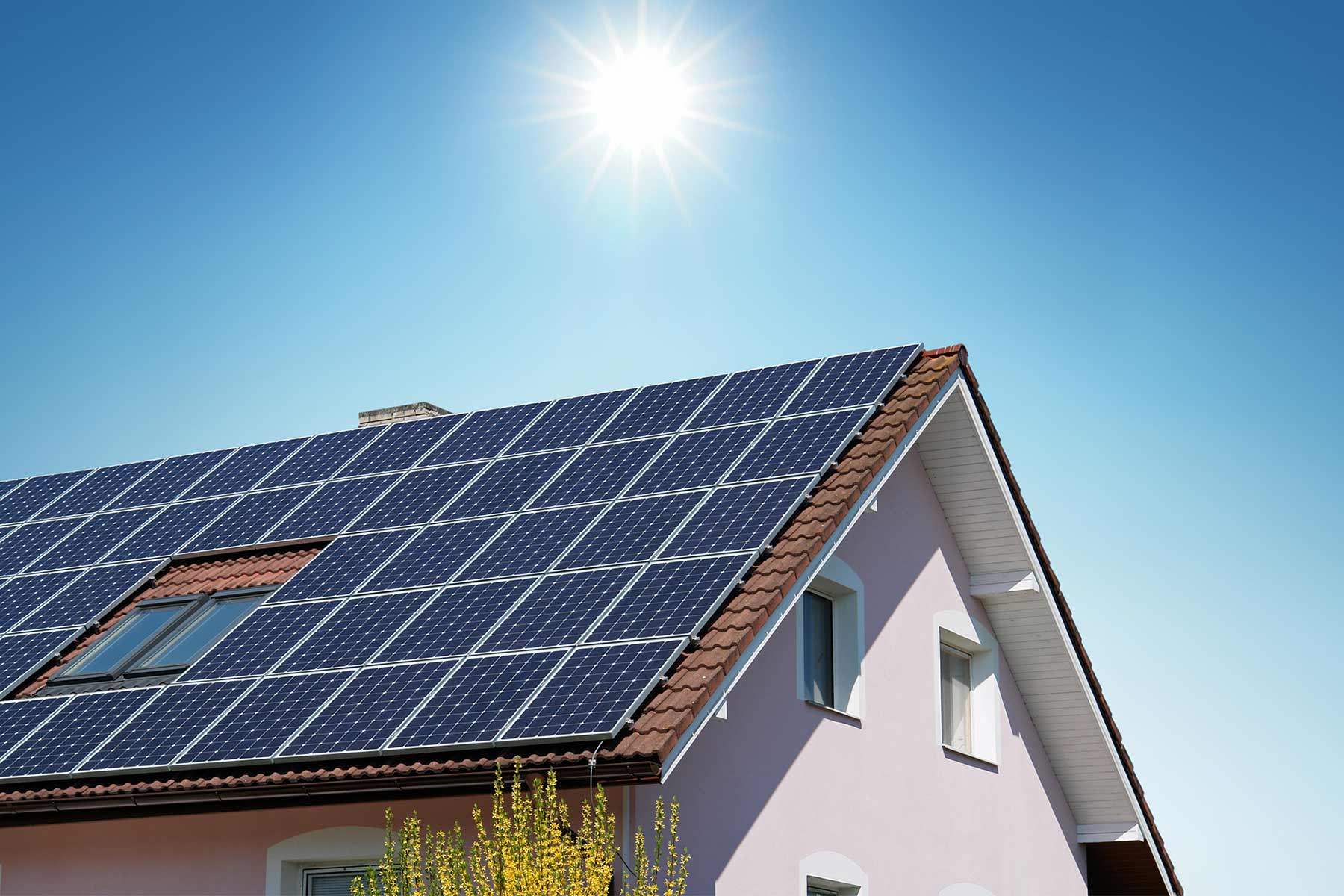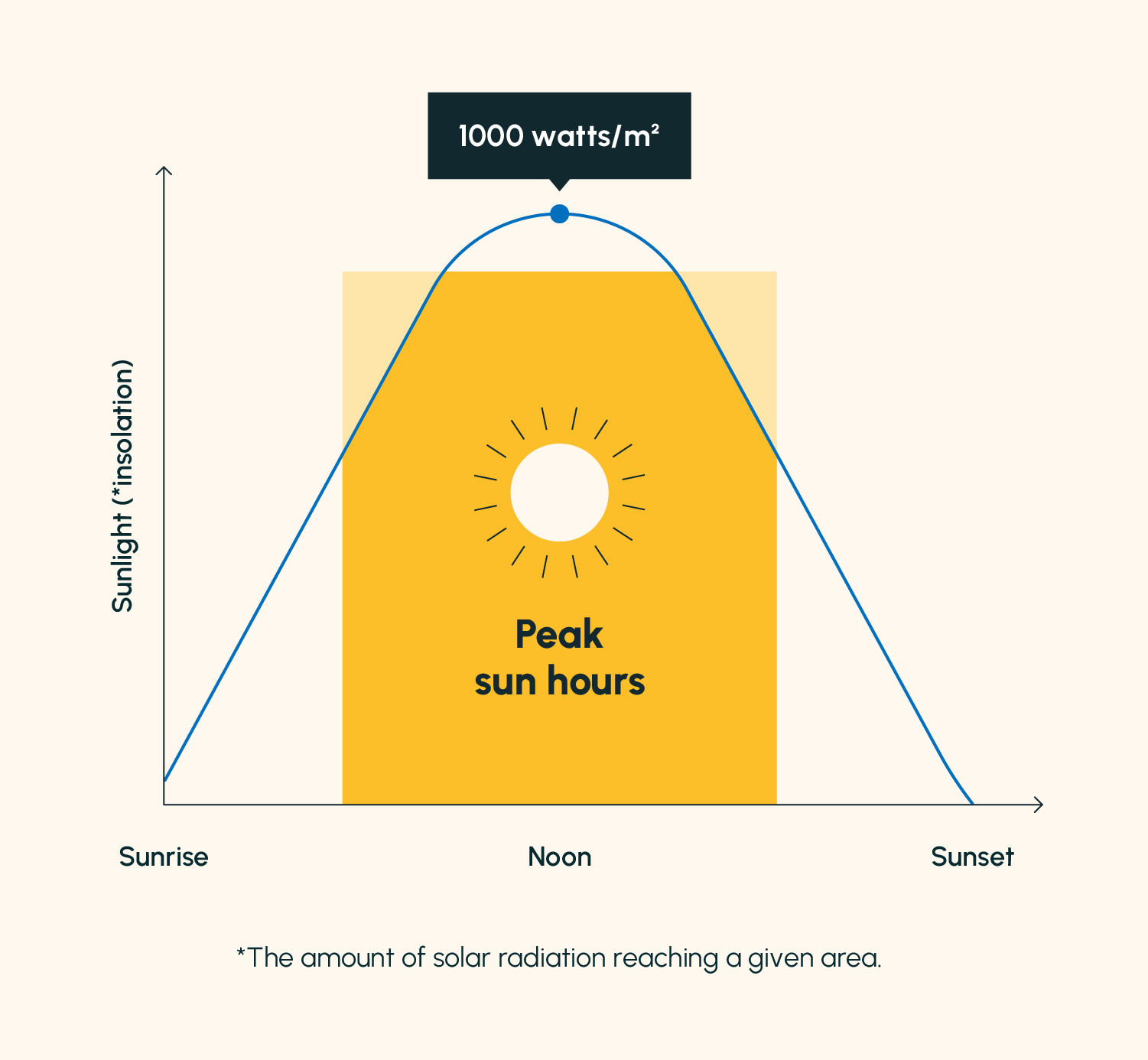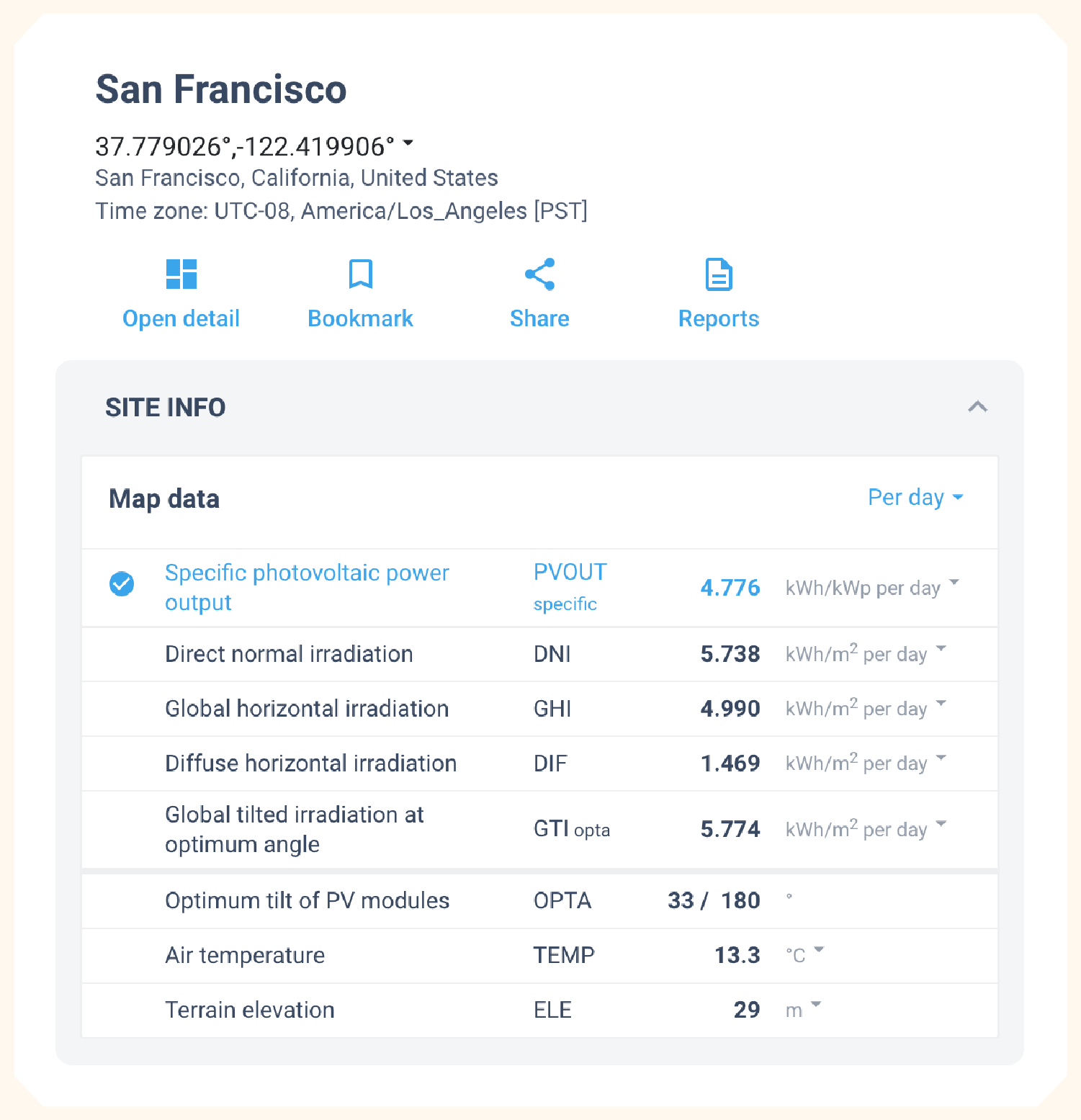# How Many Solar Panels Do I Need for 2,000 kWh per Month? System Sizing by Location

Depending on how much sunlight your home receives and the efficiency of your solar panels, you will need anywhere between 25 and 65 solar panels to produce 2,000 kilowatt-hours (kWh) per month.

For homes with relatively high electricity usage that plan to rely entirely on solar energy, it's imperative to properly size your system and purchase the correct number of solar panels to meet your needs. Unfortunately, there isn't a straightforward answer — the number of panels you need depends on a lot of factors including panel wattage, your system's location, panel angle, and more.

Specifically, if you're wondering how many solar panels you need to produce 2,000 kWh per month, this number can range from 25 to 65 panels. If you live in California and use very efficient panels, you'll need closer to 25 panels. If you live in Washington and use less efficient panels, you'll need closer to 65. In this post we'll walk through how to determine exactly where you fall in this range.

## Calculating a Number of Panels Based on Kilowatt-Hours and Sun Hours

The number of solar panels you need depends on several factors — most notably, your location. This is because Global Irradiation (GI) — better known as sunlight — varies significantly by location.

### Quick Refresher on Kilowatt-Hours (kWh)You probably understand the gist of kilowatt-hours — after all, you're reading this post for a reason — but let's review what it means and how we calculate it.

Kilowatt-hours (kWh) is the unit used to measure electrical energy. One kWh encompasses 1,000 watt-hours (Wh).

You'll hear solar panel systems measured in kilowatts (kW), however. Kilowatts are the unit used to measure electrical power — the rate that electricity flows.

You can determine kWh as long as you know the wattage of a device. Here's the equation:

Electrical energy (kWH) = Electrical power (kW) x hours

If you're unsure how much power you use on a daily basis, give our off-grid solar system calculator a spin to estimate the electrical load that your appliances consume.

Since solar panels are labeled with their wattage, you can easily determine how much electrical energy they produce over a certain period of time. For example, a 350 W (0.35 kW) solar panel running for ten hours would produce 3.5 kWh of energy.

### Quick Refresher on Peak Sun HoursPeak sun hours measures how much solar irradiance (the amount of sunlight an area receives from the sun, measured in Watts per meter squared) that a certain location receives. Solar panels need 1000 W/meter² to produce their full wattage.

Since solar irradiance does not reach 1000 W/meter² at all times of day for all areas, we need to calculate an area's peak sun hours to determine how well solar panels will perform in that area.

To calculate peak sun hours, multiply solar irradiance by how many hours an area receives that level of irradiance. For example, if an area receives 600 W/meter² of solar irradiance over the course of five hours, that area has received 3,000 Wh/meter² (3.0 kWh/meter²) of sunlight, or three peak sun hours. You can also find your area's peak sun hours using the Global Solar Atlas.

To determine how much energy solar panels can produce in a certain area, multiply their wattage by their peak sun hours. For example, let's say you have 3 kW worth of solar panels (maybe 10 panels each with an efficiency of 300 W) in the same area as the example above (which receives three peak sun hours). Those solar panels can produce 9 kWh of electricity within the five hours that the area receives 600 W/meter² of solar irradiance.

### The Calculation

To calculate the exact number of solar panels you need for your system, we'll lean on the model that Solargis developed for Global Solar Atlas. For this equation, you'll need your desired monthly kWh, your specific photovoltaic power output (PVOUT; more on this shortly), the power output of a single panel, and the average number of days in a month (30.4).

# of solar panels = [(Desired monthly kWh ÷ 30.4) ÷ PVOUT] ÷ kW of one solar panel

To start, search for your location with Global Solar Atlas and identify your area's daily PVOUT. This number indicates how many kWh that every kW of solar panels in a specific area can produce — essentially, how well your solar panels can achieve their full potential.

Let's practice this equation with an example. Say you're building a solar system in San Francisco using panels rated at 370 W (0.37 kW). You want these panels to generate 2,000 kWh per month. First, we'll identify San Francisco's daily PVOUT using the Global Solar Atlas (shown below).We now have all of the variables we need:

• Desired monthly kWh = 2,000 kWh
• PVOUT = 4.776 kWh/kWp per day
• Individual panel power = 0.37 kW

Here's what our equation would look like:

# of solar panels = [(2,000 kWh ÷ 30.4) ÷ 4.776 kWh/kWp] ÷ 0.37 kW

# of solar panels = (65.79 ÷ 4.776 kWh/kWp) ÷ 0.37 kW

# of solar panels = 13.78 ÷ 0.37 kW

# of solar panels = 37.24, rounded up to 38 solar panels

As you can see, several factors go into the number of solar panels you'd need to produce a certain amount of energy. If you increase the efficiency of your panels or live in an area with a higher PVOUT, you'll need fewer to reach your desired output.

## Average Number of Solar Panels Needed to Power a House in the 10 Most Populated States

If you live in one of the ten most populated states in the U.S., we ran the numbers for you to estimate your system size and how many solar panels you'd need for 1,000 and 2,000 kWh per month.

State # of panels (350 kW) needed for
1,000 kWh per month
System size (kW) # of panels (350 kW) needed for
2,000 kWh per month
System size (kW)
California 19 6.7 38 13.3
Texas 22 7.7 43 15.1
Florida 22 7.7 43 15.1
New York 28 9.8 55 19.3
Pennsylvania 27 9.5 53 18.6
Illinois 24 8.4 48 16.8
Ohio 26 9.1 51 17.8
Georgia 22 7.7 44 15.4
North Carolina 23 8.1 45 15.8
Michigan 26 9.1 52 18.2

## FAQ: Determining Solar Panel Quantity

Several factors impact the number of solar panels needed to power your house. Below, we answer some common questions about solar system sizing.

### What Size Solar System Do I Need for 1,500 kWh Per Month?

On average, you'll need about a 10 kW solar system. Depending on factors like where you live and the efficiency of your solar panels, you will likely need between a 7 kW and a 15 kW system. The lower end (7 kW, or about 14 panels) would apply if you live in California and use 500 W panels, and the higher end (15 kW, or about 60 panels) would apply if you live in Washington and use 250 W panels.

### How Much Does a 10 kW Solar System Cost?

A 10 kW solar system installation will usually cost somewhere between \$20,000 and \$30,000, depending on location and component quality. Give our solar panel cost calculator a spin to estimate how much your specific system would cost.

### How Much Power Does a 10 kW Solar System Produce?

A 10 kW solar system produces between 11,000 and 15,000 kWh per year, or 40 kWh and 55 kWh per day, depending on your location and other factors like solar panel angle.

### Is 10 kW Enough to Run a House?

Yes, a 10 kW solar system should produce more than enough energy to power an average-sized house (about 2,000 square feet).

Solartap wants to help power your home for less by offering premium, highly efficient panels with installation costs far below the competition. Get a quote today using our solar calculator.